Back

# Simple way to save up on your struct memory in golang

After going through this article, you will be taking back a simple yet an effective way to save quite a memory while simply declaring your struct. Besides you will realise that the arrangement of your struct fields matter too.

Before jumping into it, let us quickly understand some terms which will be essential to go ahead.

## Word and Word Size

A word is nothing but the amount of data that a machine can process in one CPU Cycle from memory.

Let's say that the system on which you are working has a 32-bit processor. Now what does this mean?

Processors do not generally read 1 byte of data at a time from memory. It reads 1 word at a time. A 32-bit processor means that your processor can process 32 bits, i.e, 4 bytes of data, at a time(in one CPU cycle). This brings us to a conclusion that the word size of a 32-bit processor is 4 bytes.

Similarly for a 64-bit processor the word size will be 8 bytes.

## How memory is allocated to struct members in golang?[Assuming the system to be a 32-bit processor]

When an object of some structure type is declared then some contiguous block of memory will be allocated to structure members.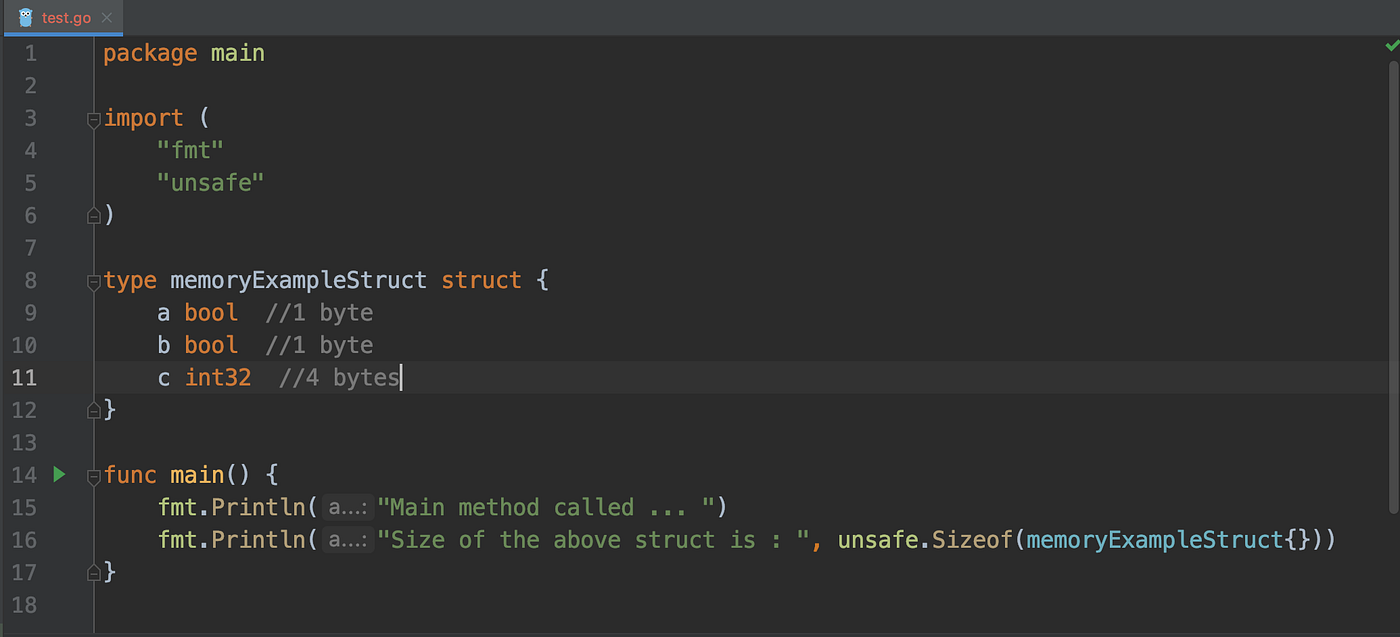Let us look at the above code.

In the above code we created a struct memoryExampleStruct and trying to print its size. So as per the above mentioned definition of memory allocation, the size of the struct should have been 1 + 1 + 4 = 6 bytes.

Well if you guessed it then let me tell you the output is not 6 bytes.The output of the above code will be 8 bytes. How is this possible now?

This is when structure padding comes into the picture which is handled itself by golang's compiler.

First let us understand what happens when the memory is calculated in a normal non-padding way.

As you all know by now that your processor will not read 1 byte of memory rather it will read 4 bytes(1 word) of memory in one CPU cycle.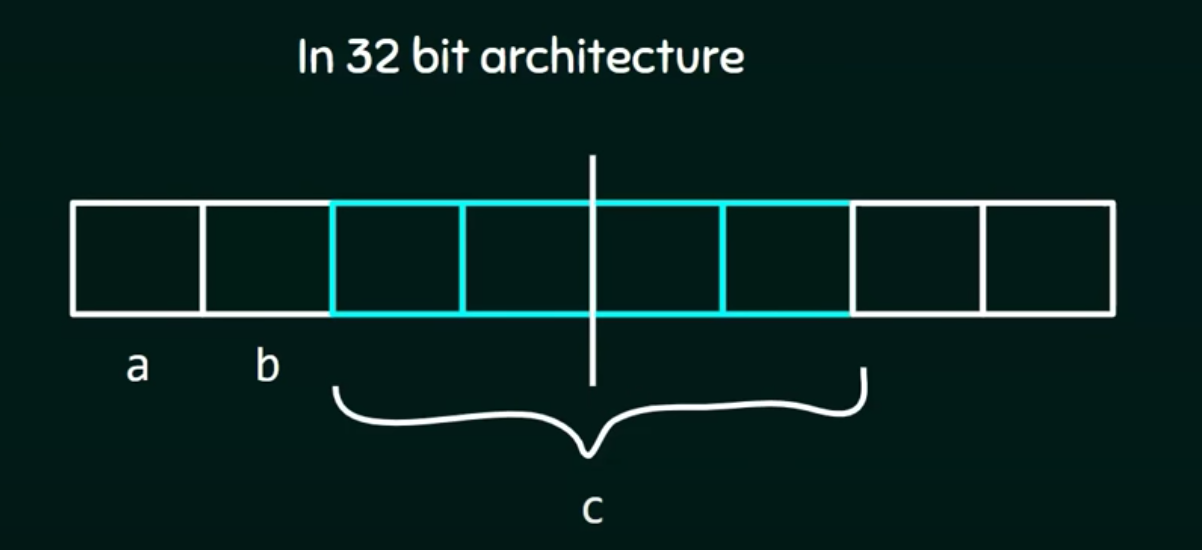Now let say your processor is reading bytes. In the first cycle it will read 4 bytes of data. Hence variable 'a'(1 byte), 'b'(1 byte) and 2 out of the 4 bytes of the variable 'c' will be processed in the first CPU cycle. And in the second CPU cycle the remaining bytes of variable 'c' will be processed.

Assume that someone wants to access the variable 'c'. For accessing 'c' processor has to spend 2 CPU cycles for processing a single variable. This is an unnecessary waste of CPU cycles.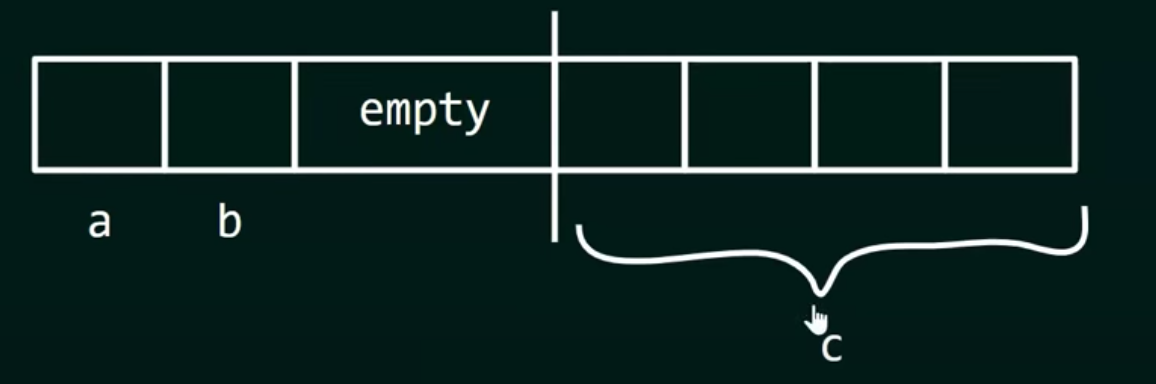So in order to avoid such wastage of CPU cycles, golang's compiler creates a padding. It allocates 2 bytes of empty space after variable 'a' and 'b'. Due to this the overlapping of variable 'c' is avoided and the variable falls into a separate CPU cycle. Though the memory processed will be more but the number of CPU cycles will be less. Now for accessing variable 'c' only 1 CPU cycle will be used.

Now what will happen if the order of the struct is rearranged something like below? Will the output be the same or more?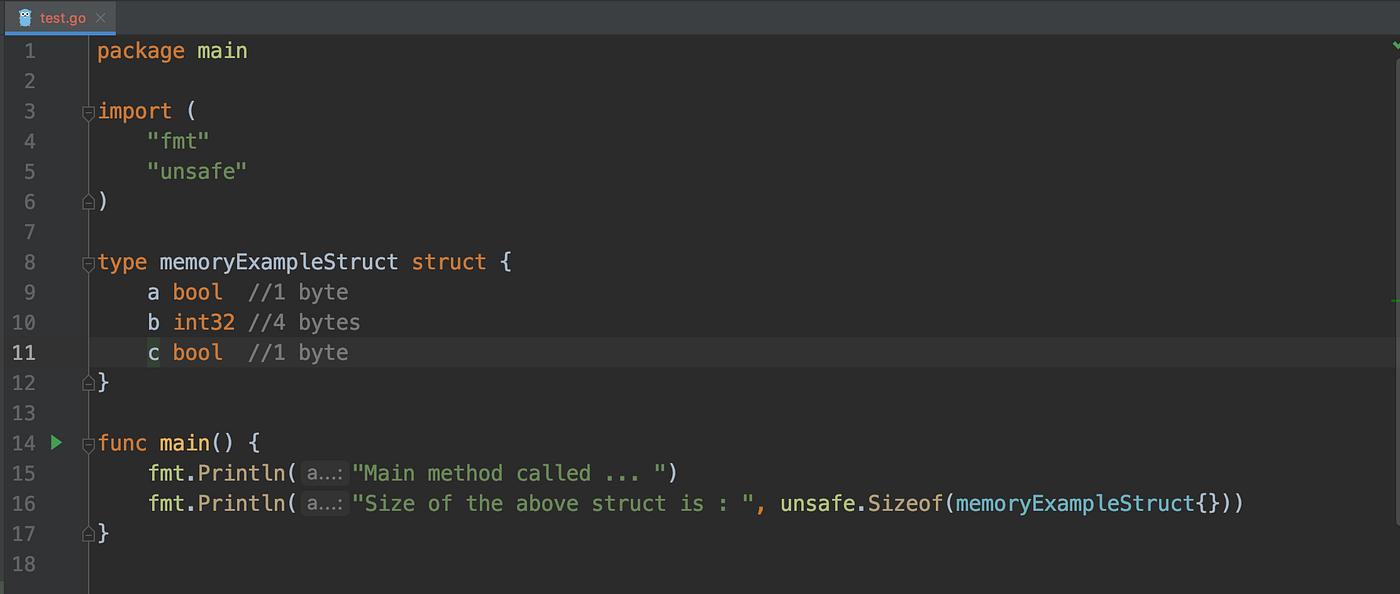The output of the above piece of code is 12. Now how does this happen?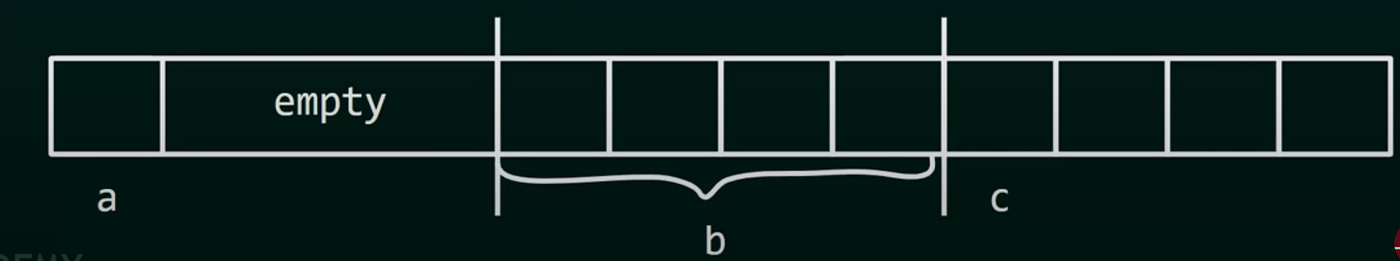Now as the order is rearranged, 'a' will be allocated 1 byte. Then we have variable 'b' which is of type int. Hence to avoid overlapping and wastage of CPU cycle, 'b' is processed in the next cycle and so on. Also if you observe 'c' of type boolean is of 1 byte but still 4 bytes will get processed. This, as I mentioned earlier, is because the processor will access 4 bytes(1 word) at a time irrespective of the variable size.

Hence it will process 3 words of memory. Which brings us to the result of 12 bytes.

## Conclusion - How to save up on memory?

As we saw in the above two examples that when the struct was arranged in an increasing order of the size of the variable then the output was 8 bytes. And when the fields of the same struct was arranged in an unorderly way then the output was 12 bytes. 4 bytes more than the previous one.

Now imagine in the above example there were only 3 fields and the padding difference was 4 bytes. Assume yourself working in a big project where let say you have defined a product model struct with more than 20 fields. Then memory wastage will be more.

Hence, it is advisable that the struct fields should be ordered in a way that it falls from highest memory at the top and lowest at the bottom as much as possible. Example- float64 or int64 at the top and bool at the bottom.

This will push all the padding(if exists) down, hence the memory wastage can be cut down.